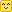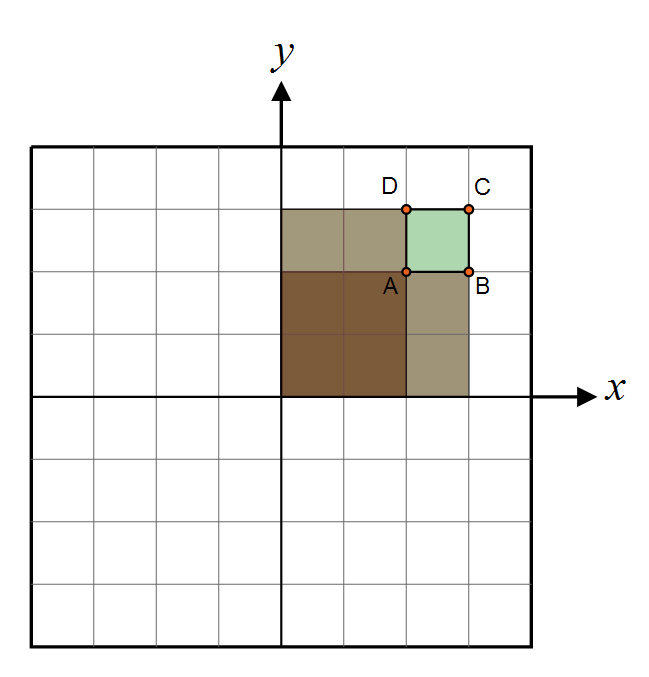Hi!

But maybe you need the solution to another, similar problem that consists in finding the solid angle of a pixel lying in the z=0 plane and orthogonally projected onto the hemisphere, but couldn't find a page with that computation?

Well let me help you with that!First of all, the document that was used initially to do the computations -- whether it be in AMD's cube map generator, Rory Driscoll's summary or this page -- is the very interesting thesis by Manne Öhrström.

## Cubemap Projection Configuration

The configuration for a cube map is that pixels are lying on a plane z=1 such as $\mathbf{p'}=(x,y,1), -1<x<1, -1<y<1$ and we project back onto the unit hemisphere by "normalizing" the vector $\mathbf{p}=\frac{\mathbf{p'}}{|\mathbf{p'}|}=\frac{(x,y,1)}{\sqrt{1+x^2+y^2}}$ as shown on the figure below:

The idea is to compute the area of a small element of surface on the hemisphere as we make it vary on the plane, we do that by computing the partial derivatives of $\mathbf{p}$ along x and y that give us the vectors $\frac{\partial \mathbf{p}}{\partial x}$ and $\frac{\partial \mathbf{p}}{\partial y}$.

As it is well-known to shader programmers, computing the cross product of these vectors gives us the normal to the sphere at this position $x, y$ on the plane, and the length of that normal is the tiny area element $dA$ on the hemisphere.

Integrating this operation (cross product and norm computation) over an interval $[a,b]\in\mathbb{R}^2$ yields:

$A(x,y) = \int_0^y \int_0^x \left | \frac{\partial \mathbf{p}}{\partial x} \times \frac{\partial \mathbf{p}}{\partial y} \right | \, da \, db \\ = \int_0^y \int_0^x (1+a^2+b^2)^{-\frac{3}{2}} \, da \, db \\ = \left | atan( \frac{xy}{\sqrt{1+x^2+y^2}} ) \right | \quad \quad \quad (1)$

It's easy to notice that $A(1,1) = \frac{\pi}{6}$ which is the solid angle covered by a quarter of our cube map face, the solid angle of an entier face would be $\frac{2\pi}{3}$ and all 6 faces of the cube map are then covering a proper solid angle of $4\pi$ and all is well!

Now, for our little problem...

## Our Projection Configuration

Our configuration is a little bit different as our points $\mathbf{p'}(x,y) = (x,y,0)$ and we project onto the hemisphere to obtain $\mathbf{p}(x,y) = (x,y,\sqrt{1-x^2-y^2})$.

The partial derivatives along x and y give:

$\frac{\partial \mathbf{p}}{\partial x} = \begin{pmatrix} 1 \\ 0 \\ -\frac{x}{\sqrt{1-x^2-y^2}} \end{pmatrix}$

$\frac{\partial \mathbf{p}}{\partial y} = \begin{pmatrix} 0 \\ 1 \\ -\frac{y}{\sqrt{1-x^2-y^2}} \end{pmatrix}$

The cross product of these 2 vectors gives:

$\frac{\partial \mathbf{p}}{\partial x} \times \frac{\partial \mathbf{p}}{\partial y} = \frac{1}{\sqrt{1-x^2-y^2}} \begin{pmatrix} x \\ y \\ \sqrt{1-x^2-y^2} \end{pmatrix}$

And the norm is:

$\left | \frac{\partial \mathbf{p}}{\partial x} \times \frac{\partial \mathbf{p}}{\partial y} \right | = \frac{1}{\sqrt{1-x^2-y^2}}$

Which looks kinda nice! Integrating where it is definite (i.e. with x and y in the unit circle) $\int_0^1\int_0^1 \frac{1}{\sqrt{1-x^2-y^2}} dx \, dy = \frac{\pi}{2}$ which is a quarter of the entire hemisphere's solid angle $2\pi$, so we're good!

Except the primitive of this expression is a bit of nightmare after all, but this is our final result:

 $A(x,y) = \int_0^y \int_0^x \frac{1}{\sqrt{1-a^2-b^2}} da \, db = \tan ^{-1}\left(\frac{x}{\sqrt{-x^2-y^2+1}}\right)+x \tan ^{-1}\left(\frac{y}{\sqrt{-x^2-y^2+1}}\right)+\frac{1}{2} \left(\tan ^{-1}\left(\frac{-x-y^2+1}{y \sqrt{-x^2-y^2+1}}\right)-\tan ^{-1}\left(\frac{x-y^2+1}{y \sqrt{-x^2-y^2+1}}\right)\right) \quad \quad \quad (2)$

I tried to further out the simplification of the last 2 atan terms since a difference of atan gives a single atan but it turns out it shows some precision issues.

## How to use this?

As explained by Driscoll, we compute the area of a single pixel by doing the exact same operation as with Summed Area Tables, that is we compute 4 values for the area of 4 sections like shown here:Then we get: $dA(x,y) = C + A - D - B$

NOTE:

• Obviously, you have to be very careful not to use eq. (2) outside of its region of definition $x^2+y^2 < 1$
• Trying to compute the hemisphere's area using eq. (2) turns out to be converging super slowly: even with a quarter disc split into 10000x10000 pixels, I can only reach 1.5535995614989679...